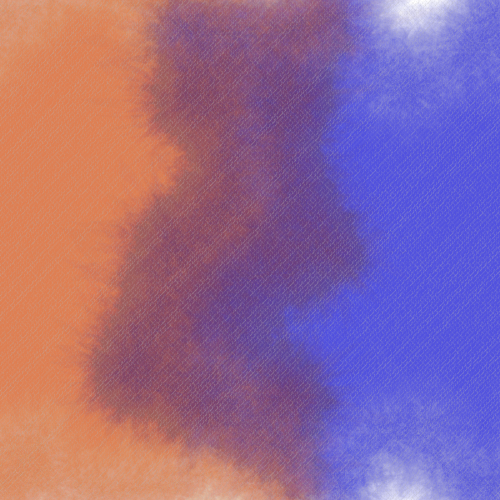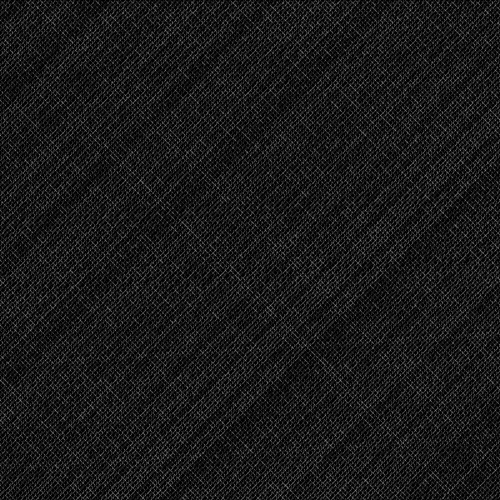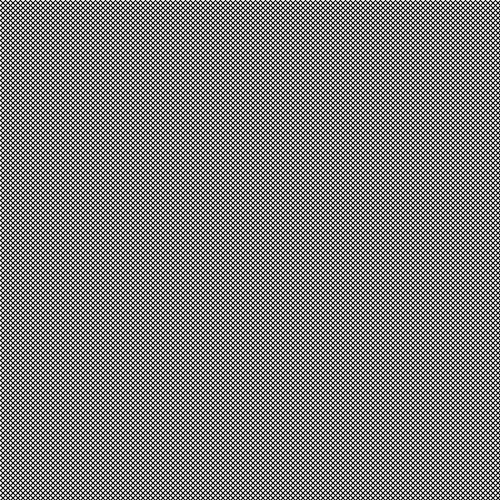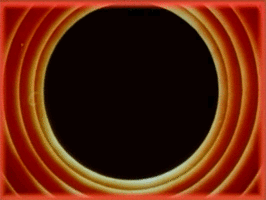# Simulating a Canvas/Denim Texture in Processing

This is another quick tip from an earlier post on how to add a canvas- or denim-like texture onto your images. The basic idea is simple: create a pattern of some kind and overlay it at a very low opacity over your final artwork. This adds a bit of texture to it, darkening and lightening parts of the image under the overlay. Here is the effect from my earlier post.Here’s the canvas texture I came up with. (It’s been drawn on a black background here for visibility as the lines are colored with a low-opacity white.)This was done by drawing two sets of lines at 45° and 135° degrees. With a low spacing between the lines, giving a nice crosshatch.Here’s the code for generating this.

void grid() {
float spacing = 5;
for (int i = -width; i < height + width; i+=spacing)
line(i, 0, i + height, height);
for (int i = height + width; i >= -width; i-=spacing)
line(i, 0, i - height, height);
}


To make things a bit more interesting, I wrote a custom line-drawing function that draws the exact same lines, but in short segments, while varying the opacity, thickness, and orientation of each one (the last one only to a small extent). Here’s the code for that.

void gridline(float x1, float y1, float x2, float y2) {
float tmp;
/* Swap coordinates if needed so that x1 <= x2 */
if (x1 > x2) { tmp = x1; x1 = x2; x2 = tmp; tmp = y1; y1 = y2; y2 = tmp; }

float dx = x2 - x1;
float dy = y2 - y1;
float step = 1;

if (x2 < x1)
step = -step;

float sx = x1;
float sy = y1;
for (float x = x1+step; x <= x2; x+=step) {
float y = y1 + step * dy * (x - x1) / dx;
strokeWeight(1 + map(noise(sx, sy), 0, 1, -0.5, 0.5));
line(sx, sy, x + map(noise(x, y), 0, 1, -1, 1), y + map(noise(x, y), 0, 1, -1, 1));
sx = x;
sy = y;
}
}


The above is a simple line algorithm that calculates the change in X and Y directions for a given step and draws a smaller line with slight variations in the position and stroke thickness. We simply replace the calls to line() in our grid() function to gridline() calls and vary the opacity randomly like shown below.

void grid() {
float spacing = 5;
for (int i = -width; i < height + width; i+=spacing) {
stroke(255, random(20, 50));
gridline(i, 0, i + height, height);
}
for (int i = height + width; i >= -width; i-=spacing) {
stroke(255, random(20, 50));
gridline(i, 0, i - height, height);
}
}


And that’s it!

This approach as a whole is fairly simple and adds a lot of depth to an image. You can color the lines to give a tinge to your image in addition to the texture, or you can try different patterns and shapes to simulate other interesting features!